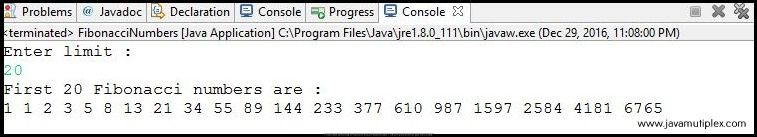## Problem Description :

Write a program in java that prints first N Fibonacci terms.

## Concept :

In Fibonacci sequence every number after the first two terms is the sum of the two preceding terms and it is defined by following recurrence relation.
F(N)=F(N-1)+F(N-2)
For example - First 10 Fibonacci terms are 1,1,2,3,5,8,13,21,34,55.

## Output :Output - How to generate first n Fibonacci terms in Java?

## References :

Thank you friends, I hope you have clearly understood the solution of this problem. If you have any doubt, suggestion or query please feel free to comment below. You can also discuss this solution in our forum.

Tags : Fibonacci terms in Java, Solution in Java,  Dynamic programming, for loop, if else statement.How to get first N Fibonacci terms in Java?Reviewed by Rohit Agarwal on 12/29/2016 Rating: 5